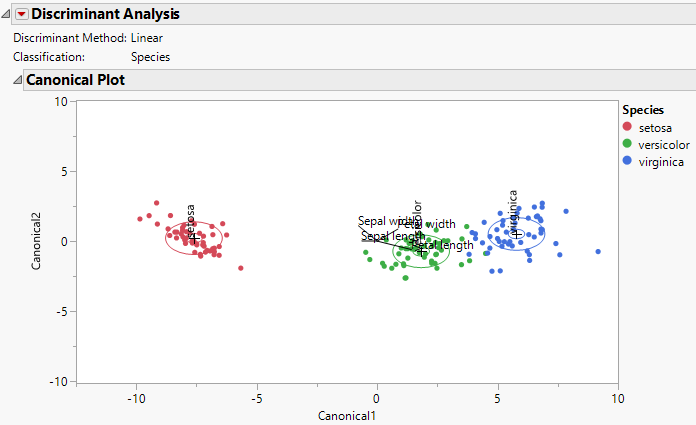Multivariate Methods > Discriminant Analysis
Publication date: 11/10/2021

# Discriminant Analysis

##### Predict Classifications Based on Continuous Variables

Discriminant analysis predicts membership in a group or category based on observed values of several continuous variables. Specifically, discriminant analysis predicts a classification (X) variable (categorical) based on known continuous responses (Y). The data for a discriminant analysis consist of a sample of observations with known group membership together with their values on the continuous variables.

For example, you might attempt to classify loan applicants into three loan categories (X) based on expected profitability: low interest rate loan, long term loan, or no loan. You might use continuous variables such as current salary, years in current job, age, and debt burden, (Ys) to predict an individual’s most profitable loan category. You could build a predictive model to classify an individual into a loan category using discriminant analysis.

Features of the Discriminant platform include the following:

A stepwise selection option to help choose variables that discriminate well.

A choice of fitting methods: Linear, Quadratic, Regularized, and Wide Linear.

A canonical plot and a misclassification summary.

Discriminant scores and squared distances to each group.

Options to save prediction distances and probabilities to the data table.

Figure 5.1 Canonical Plot